##### Actions

(diff) ← Older revision | Latest revision (diff) | Newer revision → (diff)

The representation of a Lie groupcontragredient to the adjoint representation Ad of(cf. Adjoint representation of a Lie group). The coadjoint representation acts on the dualof the Lie algebraof the group.

Ifis a real matrix group, i.e. a subgroup of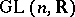, thenis a subspace of the space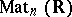of real matrices of order. Let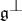be the orthogonal complement ofrelative to the bilinear formletbe some subspace ofcomplementary to, and letbe the projection ontoparallel to. Then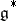is identified withand the coadjoint representation is given by the formula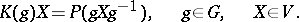The corresponding representation of the Lie algebrais also called the coadjoint representation. In the case above it is defined by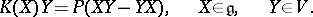The coadjoint representation plays a fundamental role in the orbit method (see ). Each-orbitin the coadjoint representation carries a canonical-invariant symplectic structure. In other words, on each orbitthere is a uniquely defined non-degenerate-invariant closed differential-form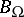(whence it follows that all-orbits in the coadjoint representation are even-dimensional). To obtain an explicit expression forone proceeds as follows. Let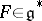, letbe the orbit through the pointand let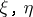be tangent vectors toat. There existandinsuch that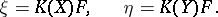Then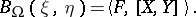For every, the vector fieldis Hamiltonian with respect to; as its generator (generating function) one can takeitself, considered as a linear function on.

The stabilizer of a point with orbit of maximal dimension in the coadjoint representation is commutative . The Poisson bracket arising on each orbit generates a single Berezin bracket, which defines the structure of a local Lie algebra (cf. Lie algebra, local), in the space of smooth functions on(see ). The coordinate expression for the Berezin bracket iswhereare the structure constants of.

How to Cite This Entry: